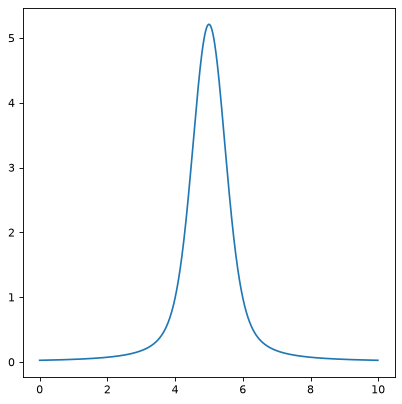# Voigt1D¶

class `astropy.modeling.functional_models.``Voigt1D`(x_0=0, amplitude_L=1, fwhm_L=0.6366197723675814, fwhm_G=0.6931471805599453, method='humlicek2', **kwargs)[source]

One dimensional model for the Voigt profile.

Parameters
x_0

Position of the peak

amplitude_L

The Lorentzian amplitude (peak of the associated Lorentz function) - for a normalized profile (integrating to 1), set amplitude_L = 2 / (np.pi * fwhm_L)

fwhm_L

The Lorentzian full width at half maximum

fwhm_G

The Gaussian full width at half maximum

method`str`, optional

Algorithm for computing the complex error function; one of ‘Humlicek2’ (default, fast and generally more accurate than `rtol=3.e-5`) or ‘Scipy’, alternatively ‘wofz’ (requires `scipy`, almost as fast and reference in accuracy).

Other Parameters
fixed`a` `dict`, optional

A dictionary `{parameter_name: boolean}` of parameters to not be varied during fitting. True means the parameter is held fixed. Alternatively the `fixed` property of a parameter may be used.

tied`dict`, optional

A dictionary `{parameter_name: callable}` of parameters which are linked to some other parameter. The dictionary values are callables providing the linking relationship. Alternatively the `tied` property of a parameter may be used.

bounds`dict`, optional

A dictionary `{parameter_name: value}` of lower and upper bounds of parameters. Keys are parameter names. Values are a list or a tuple of length 2 giving the desired range for the parameter. Alternatively, the `min` and `max` properties of a parameter may be used.

eqcons`list`, optional

A list of functions of length `n` such that ```eqcons[j](x0,*args) == 0.0``` in a successfully optimized problem.

ineqcons`list`, optional

A list of functions of length `n` such that ```ieqcons[j](x0,*args) >= 0.0``` is a successfully optimized problem.

Notes

Either all or none of input `x`, position `x_0` and the `fwhm_*` must be provided consistently with compatible units or as unitless numbers. Voigt function is calculated as real part of the complex error function computed from either Humlicek’s rational approximations (JQSRT 21:309, 1979; 27:437, 1982) following Schreier 2018 (MNRAS 479, 3068; and `hum2zpf16m` from his cpfX.py module); or `wofz` (implementing ‘Faddeeva.cc’).

Examples

```import numpy as np
from astropy.modeling.models import Voigt1D
import matplotlib.pyplot as plt

plt.figure()
x = np.arange(0, 10, 0.01)
v1 = Voigt1D(x_0=5, amplitude_L=10, fwhm_L=0.5, fwhm_G=0.9)
plt.plot(x, v1(x))
plt.show()
```

(png, svg, pdf)Attributes Summary

 `amplitude_L` `fwhm_G` `fwhm_L` `input_units` This property is used to indicate what units or sets of units the evaluate method expects, and returns a dictionary mapping inputs to units (or `None` if any units are accepted). `param_names` Names of the parameters that describe models of this type. `sqrt_ln2` `sqrt_ln2pi` `sqrt_pi` `x_0`

Methods Summary

 `evaluate`(x, x_0, amplitude_L, fwhm_L, fwhm_G) One dimensional Voigt function scaled to Lorentz peak amplitude. `fit_deriv`(x, x_0, amplitude_L, fwhm_L, fwhm_G) Derivative of the one dimensional Voigt function with respect to parameters.

Attributes Documentation

`amplitude_L` = Parameter('amplitude_L', value=1.0)
`fwhm_G` = Parameter('fwhm_G', value=0.6931471805599453)
`fwhm_L` = Parameter('fwhm_L', value=0.6366197723675814)
`input_units`
`param_names` = ('x_0', 'amplitude_L', 'fwhm_L', 'fwhm_G')

Names of the parameters that describe models of this type.

The parameters in this tuple are in the same order they should be passed in when initializing a model of a specific type. Some types of models, such as polynomial models, have a different number of parameters depending on some other property of the model, such as the degree.

When defining a custom model class the value of this attribute is automatically set by the `Parameter` attributes defined in the class body.

`sqrt_ln2` = 0.8325546111576977
`sqrt_ln2pi` = 1.475664626635606
`sqrt_pi` = 1.7724538509055159
`x_0` = Parameter('x_0', value=0.0)

Methods Documentation

`evaluate`(x, x_0, amplitude_L, fwhm_L, fwhm_G)[source]

One dimensional Voigt function scaled to Lorentz peak amplitude.

`fit_deriv`(x, x_0, amplitude_L, fwhm_L, fwhm_G)[source]

Derivative of the one dimensional Voigt function with respect to parameters.